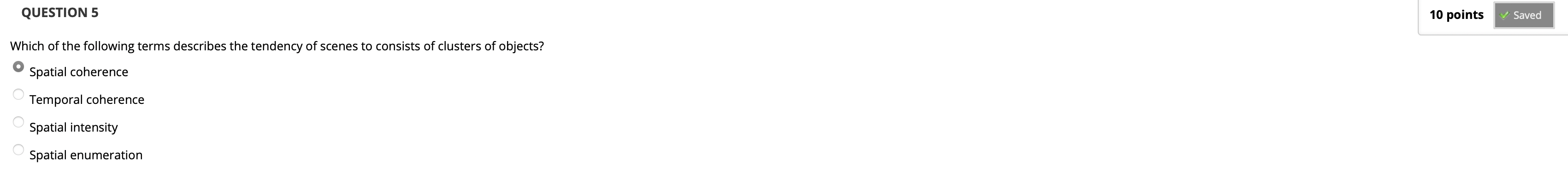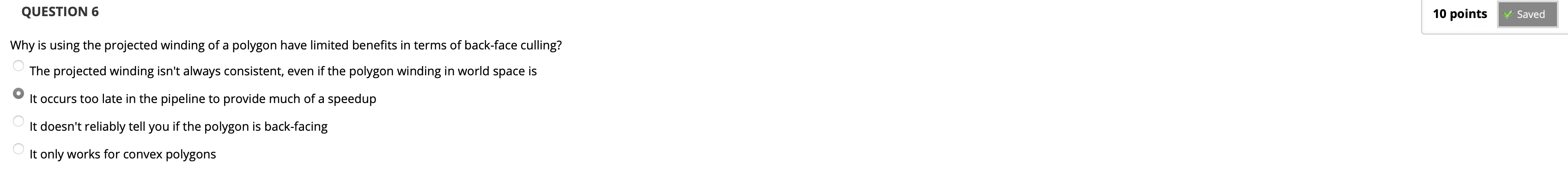# 高级计算机图形学:每周习题解析

## Solution to Weekly Quiz

Posted by R1NG on October 7, 2022 Viewed Times

# 每周习题解析

## Week I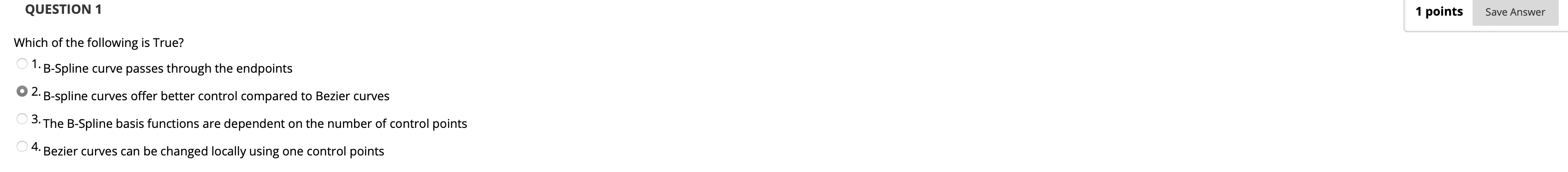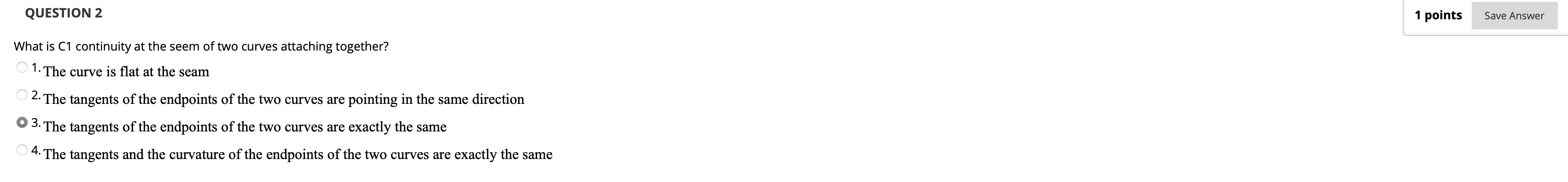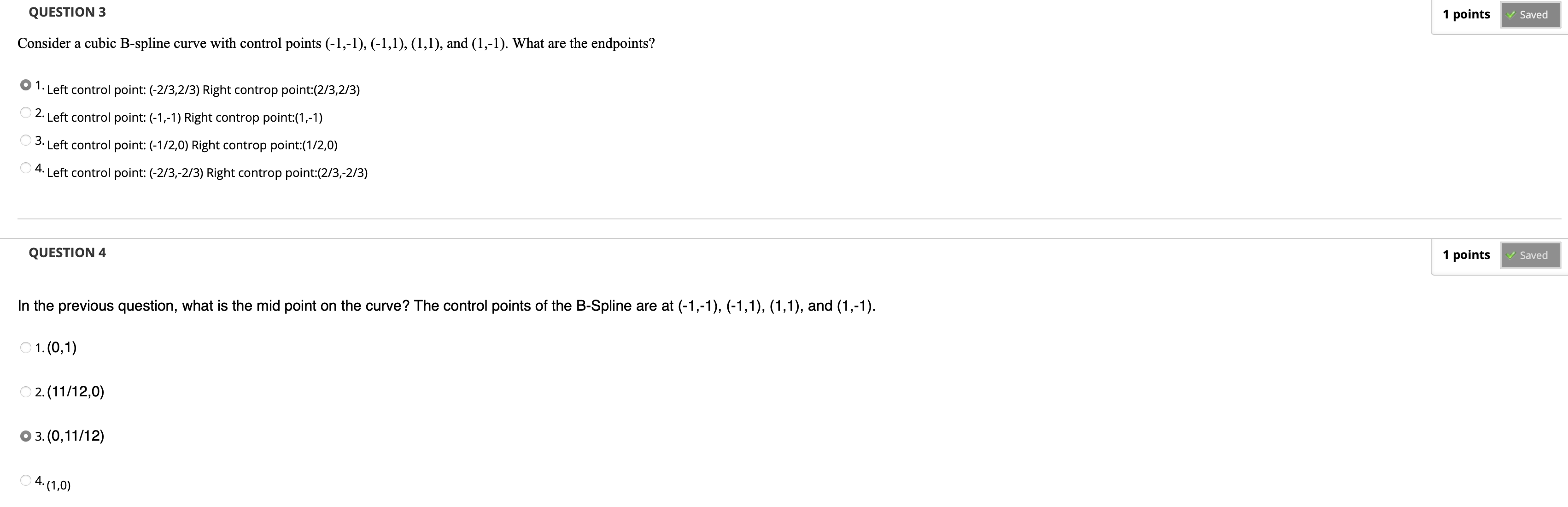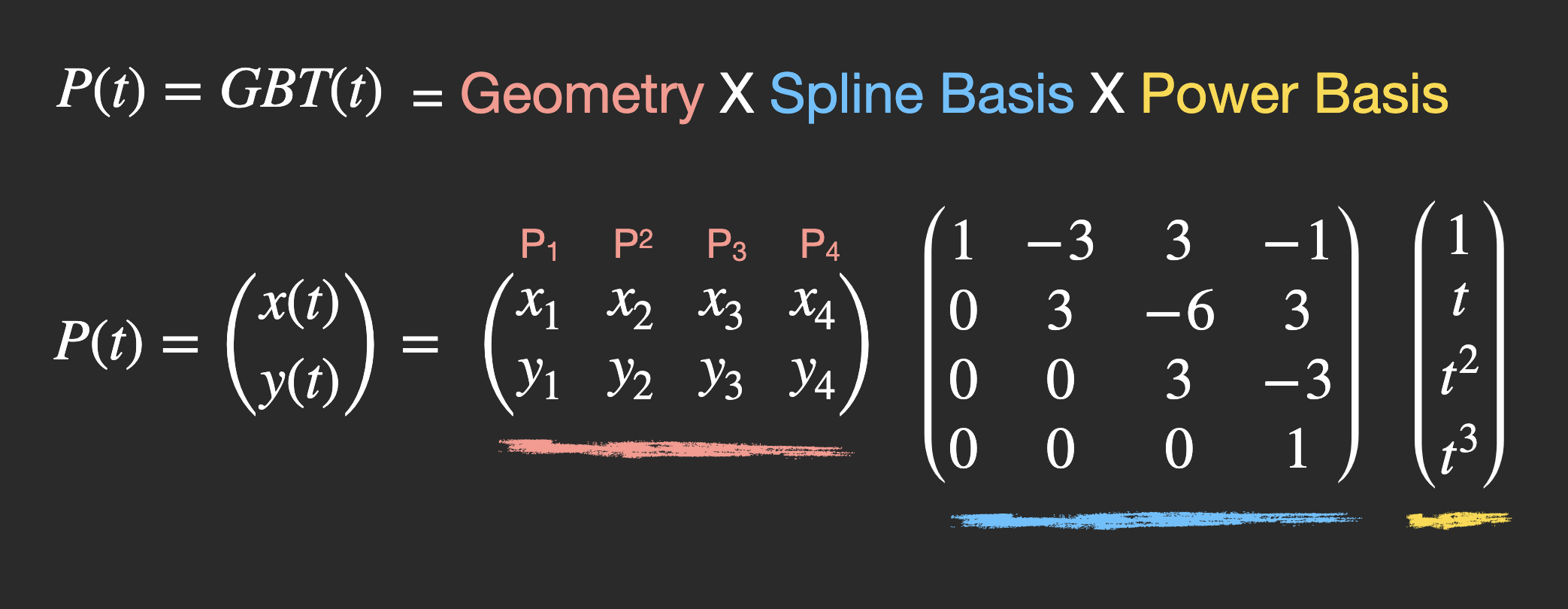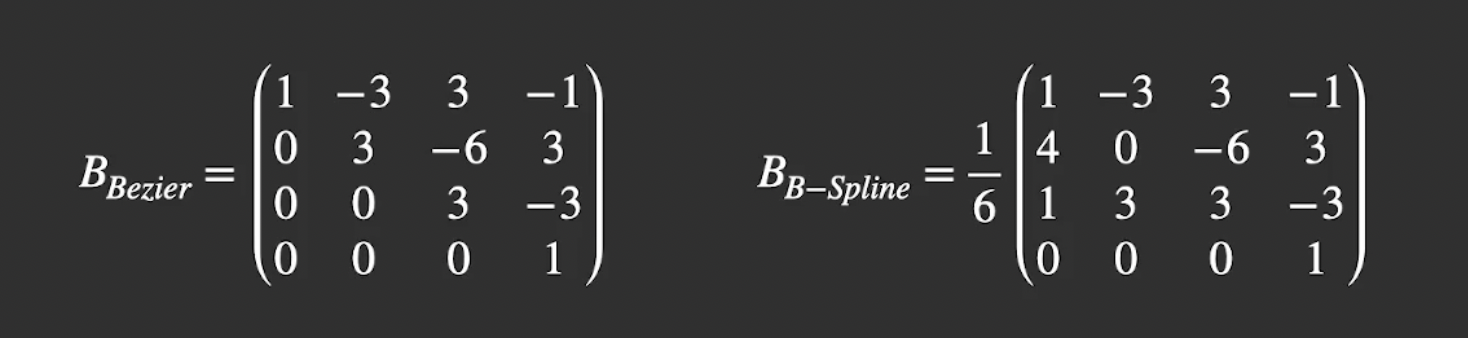$x = \frac{-4 + 6t + 6t^2 -4t^3}{6}$ $y = \frac{4 + 6t - 6t^2}{6}$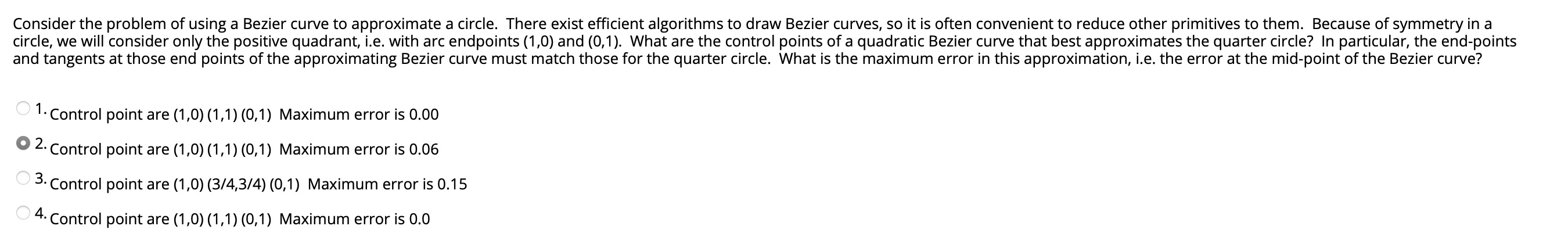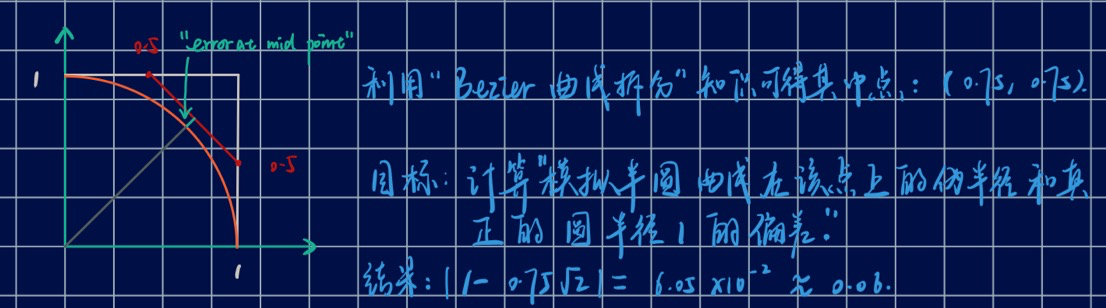## Week II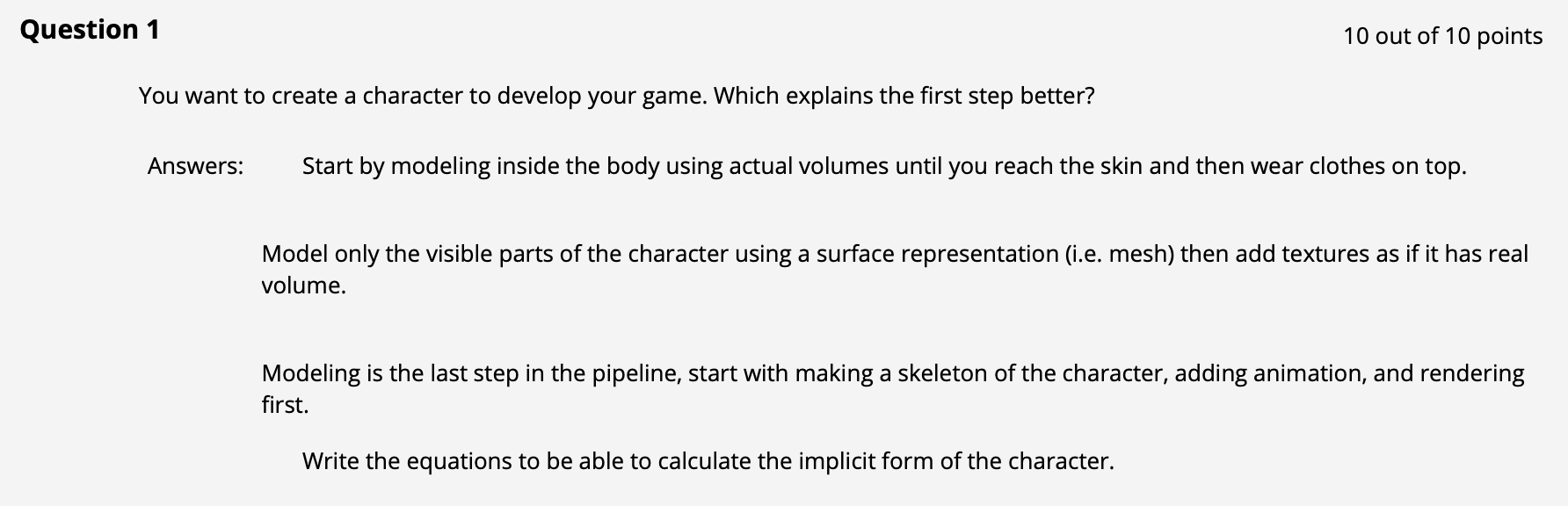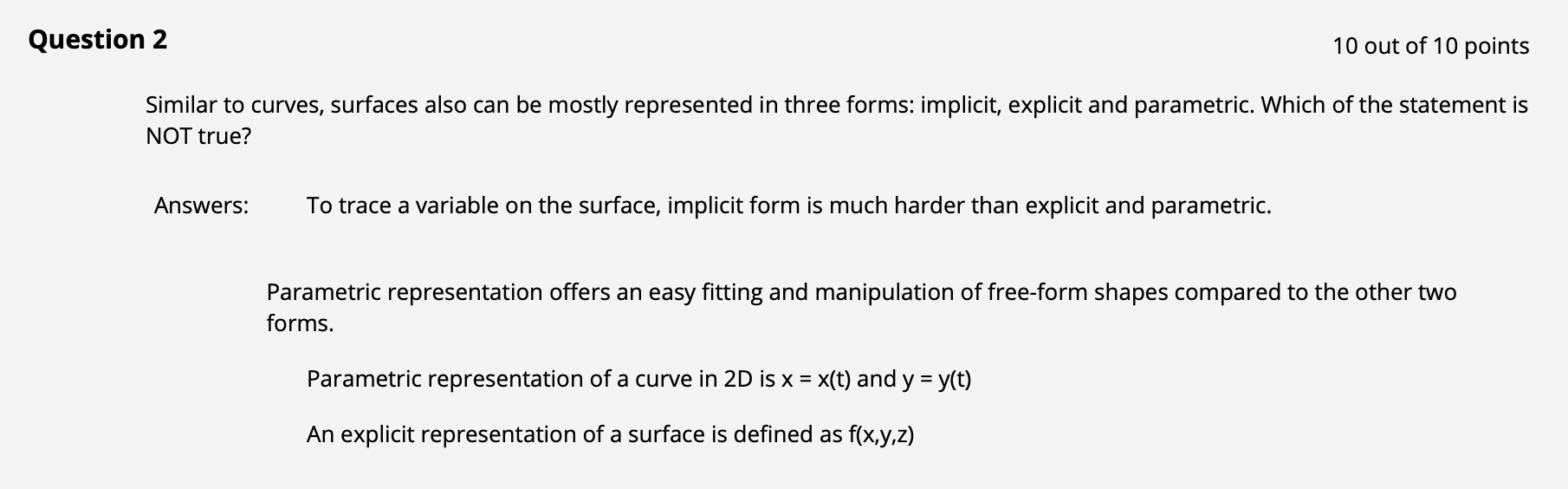A 是正确的: 由于曲线的隐式表示形如 $F(x, y, z) = 0$, 故若要 trace a variable, i.e., 给定自变量 $x, y$ 求因变量 $z$, 不同于显式表示和参数化表示的情形可以直接得出结果, 我们需要 求解隐式表示的方程 才能得到对应的 $z$.

B 也是正确的: 通过调节参数, 我们即可修改曲面的表示.

C 符合参数方程的定义, 因此也是正确的.

D 不符合显式函数表示的定义: 显式函数表示应该形如 $f(x, y) = z$.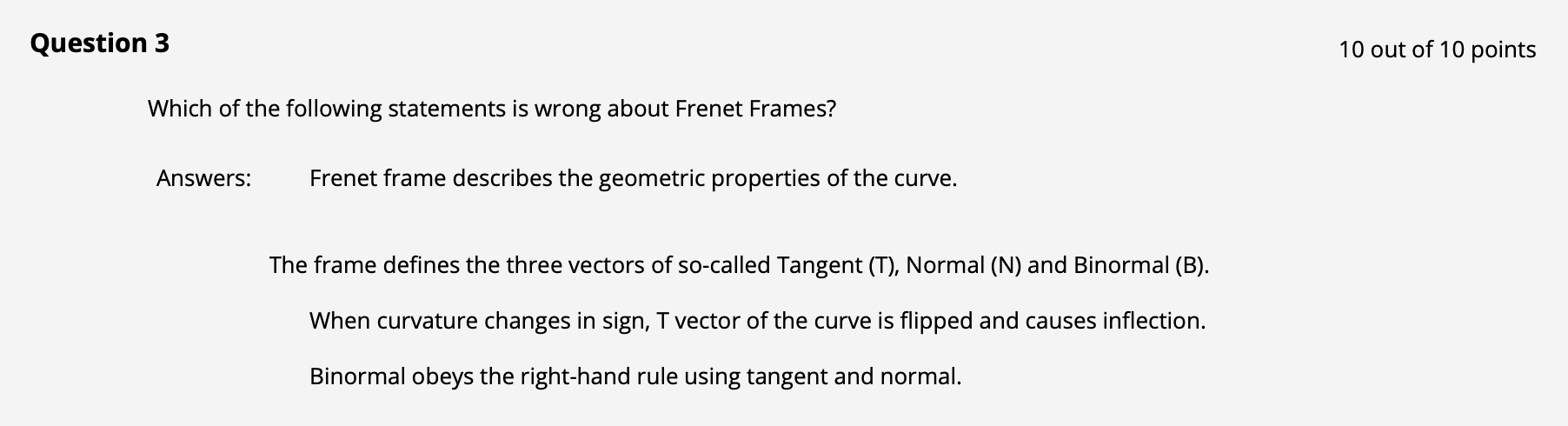A, B 是正确的: Frenet 标架的主要作用是基于曲线上的点确定关于这个点的坐标系. 回顾 Frenet 标架确定的坐标系中, 三个坐标轴分别是曲线在该点的一阶导数 切线, 二阶导数 法线 以及三阶导数 切线与法线的叉乘, 因此可以说 Frenet 标架描述了曲线的几何性质.

C 选项是错误的, 因为实际上在转折点 (Inflection Point) 处真正发生翻转的是法线 $N$, 但 这是结果而非原因. 从直接原因的角度看, $T$ 向量就是切线向量, 被曲线的一阶导数描述, 是切线向量的转折导致了法线向量的翻转.

D 选项是正确的.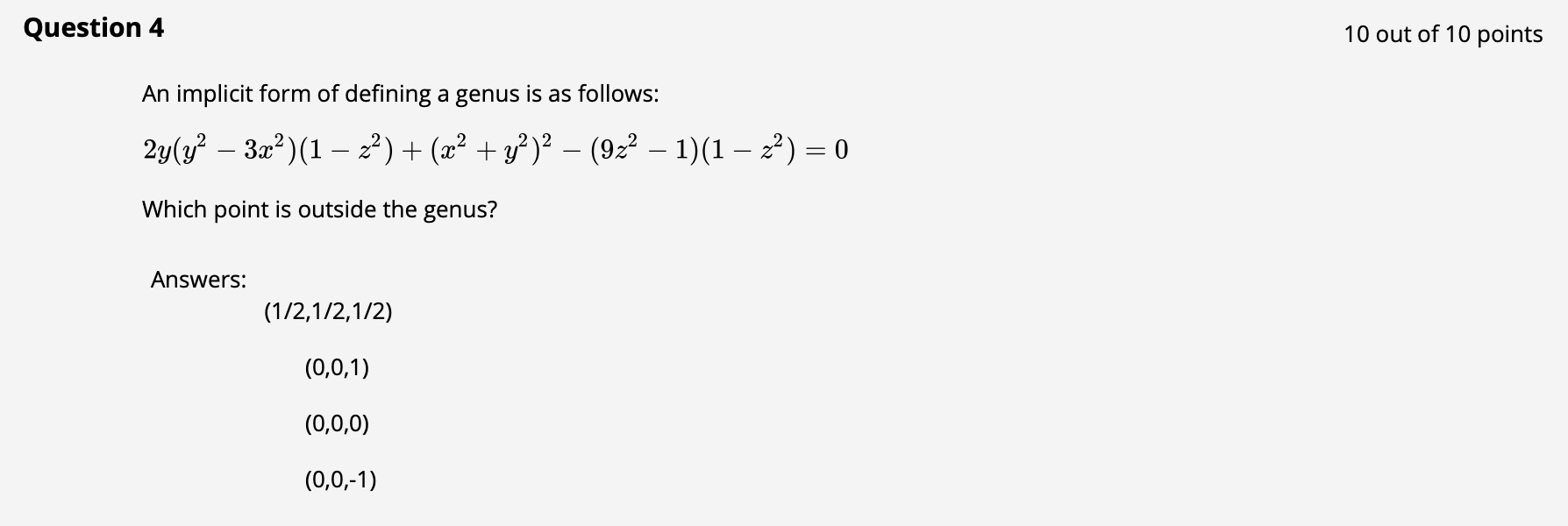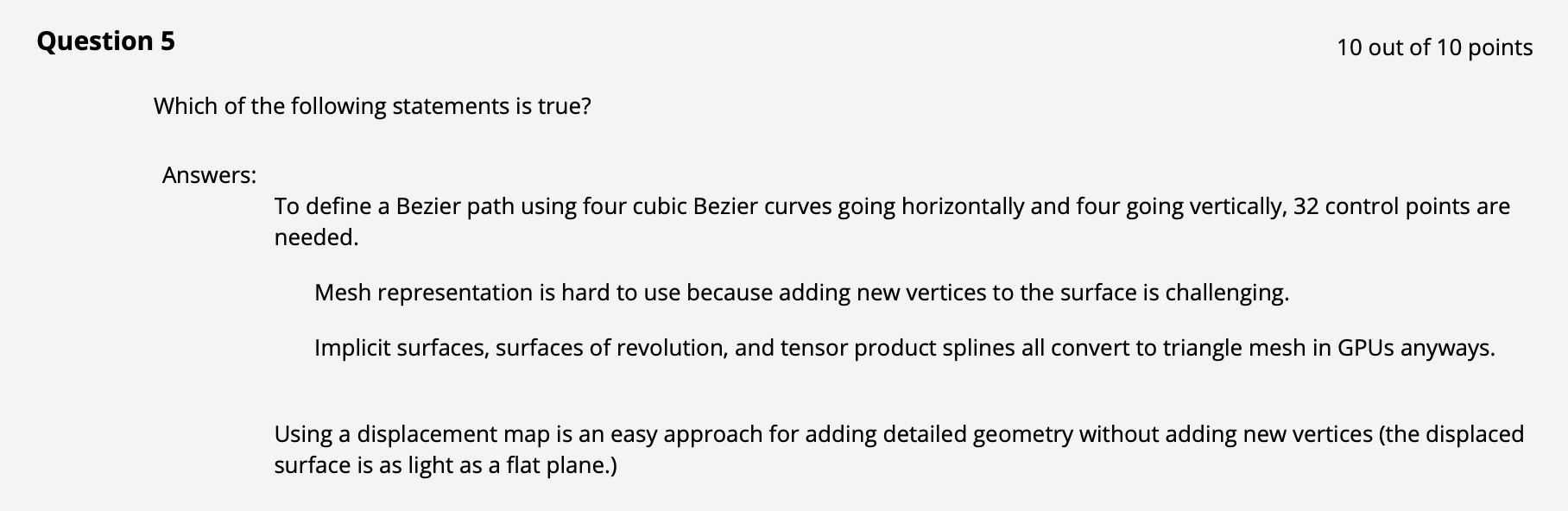A 错误: 使用双三次贝塞尔表示法表示的曲面控制点数量是 $16$ 个. B 错误: 向网格中添加新的点和边都非常方便. C 正确: 任何情况下使用任何方式建模的曲面最后在渲染管线中都会被栅格化. D 错误: Displaced Surface 是凹凸不平的, 因为平面上的点的高度会基于 Displacement Map 中存储的 offset 被抬升对应的高度, 形成 凹凸不平 的曲面.

## Week III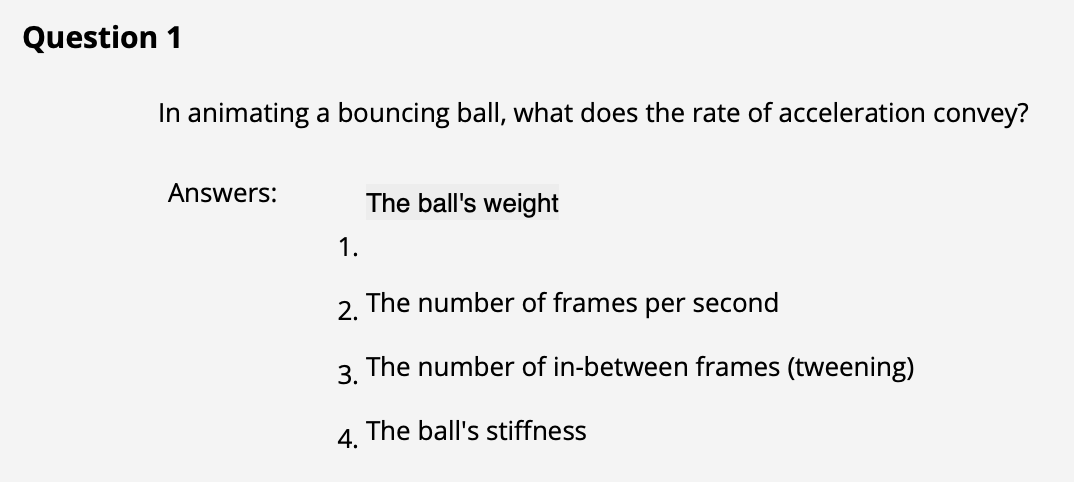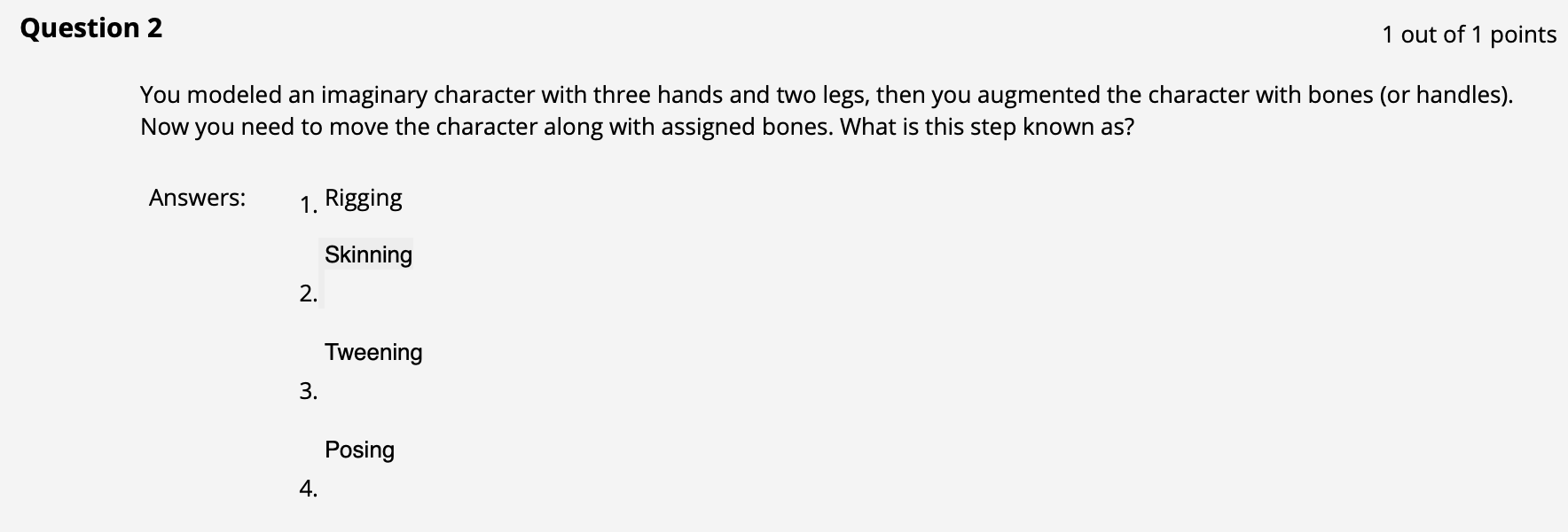Rigging: 绑定, 构造骨骼约束. Skinning: 蒙皮, 将骨骼和模型结合起来: 把 “蒙皮” 上的关键点映射到骨骼的对应位置上. Tweening: 自动插值补全关键帧间的动画, 又称补间. Posing: 将模型摆出特定的姿势.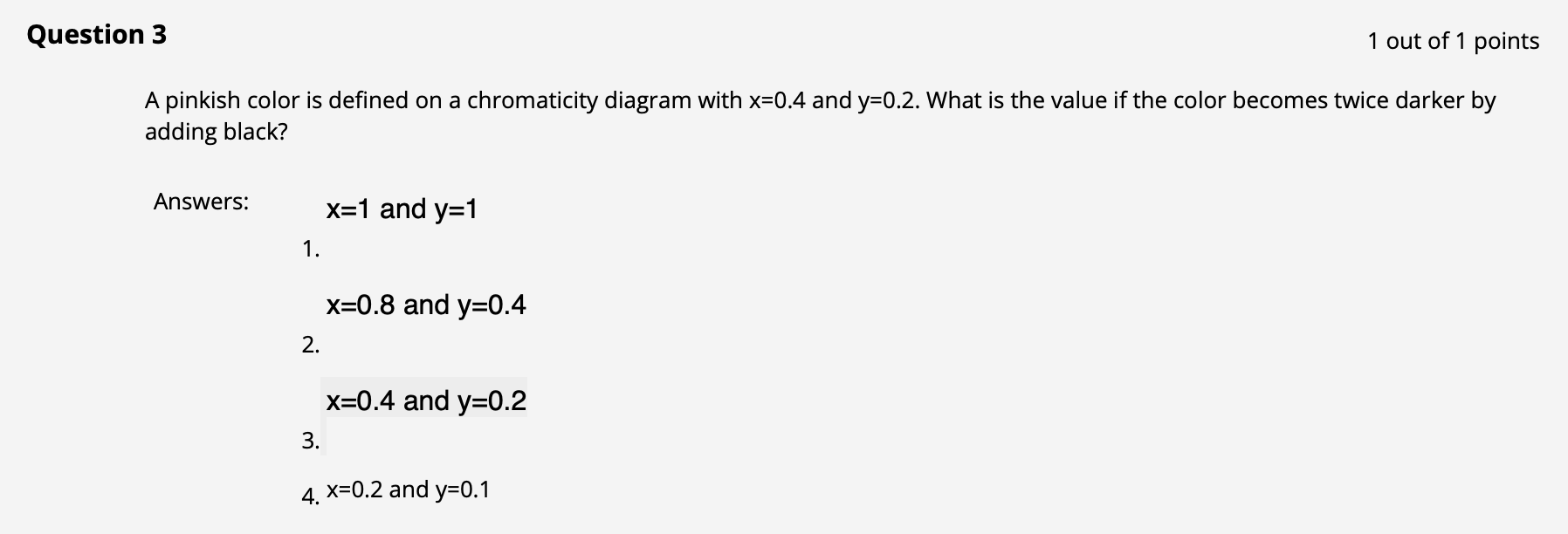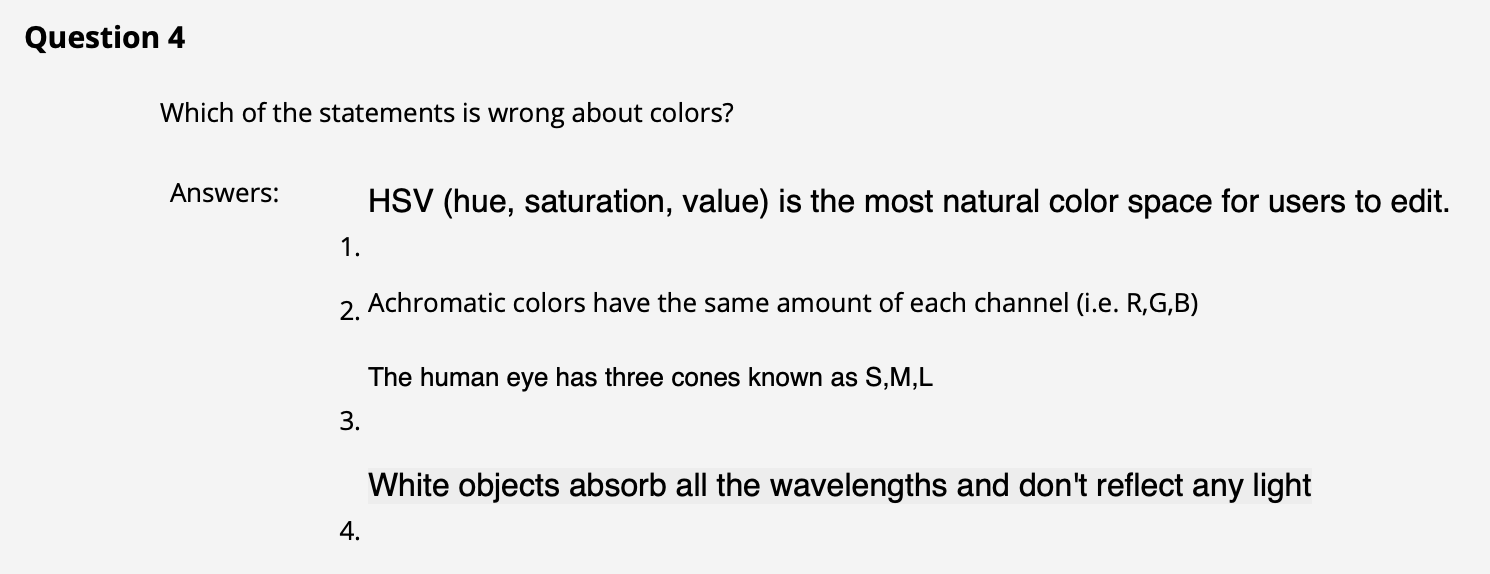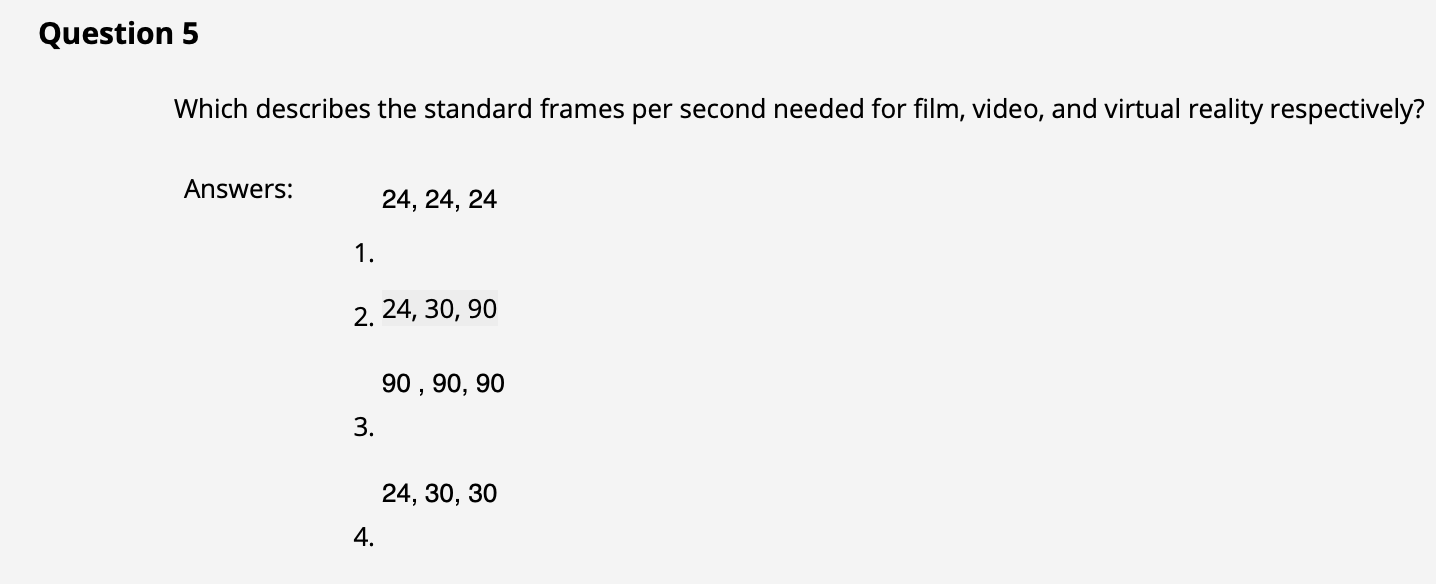## Week IV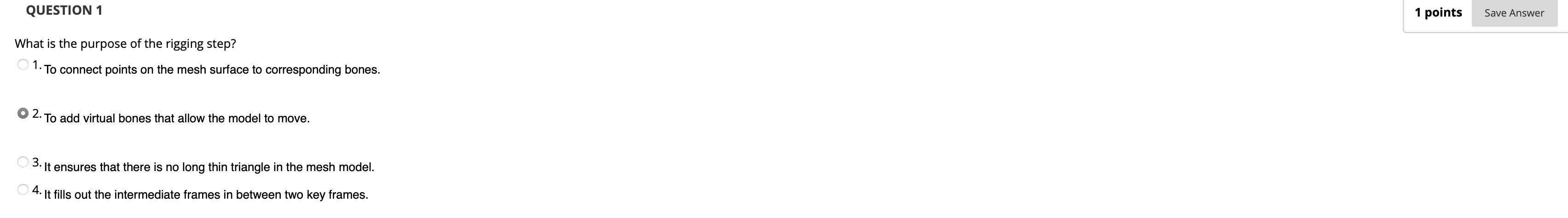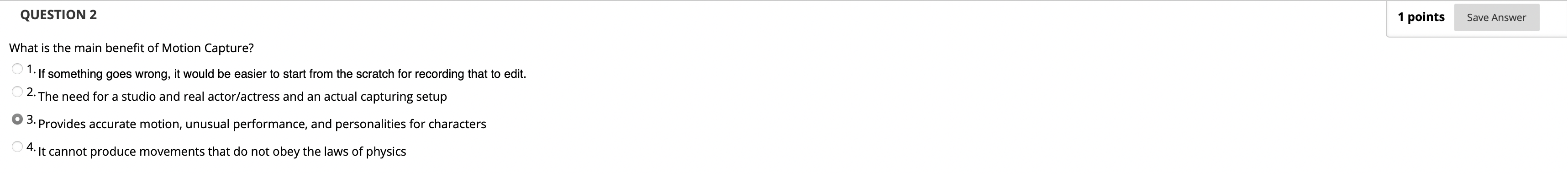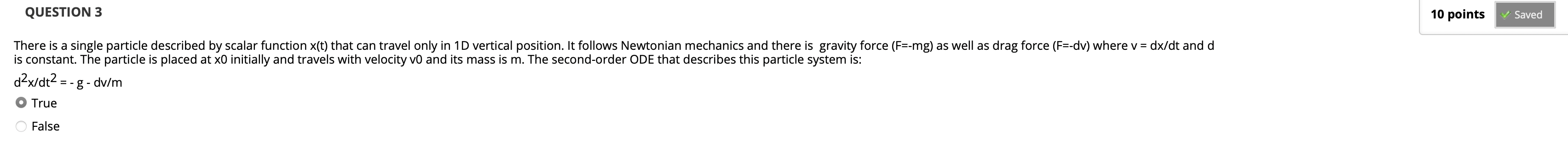$\frac{dv}{dt} = \frac{dx}{dt} \cdot \frac{d}{dt} = \frac{d^2x}{d{t^2}} = -g - \frac{dv}{m}.$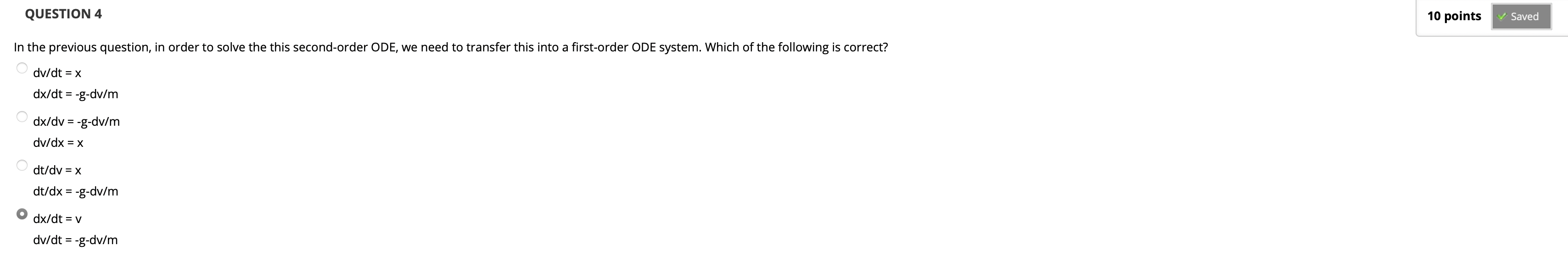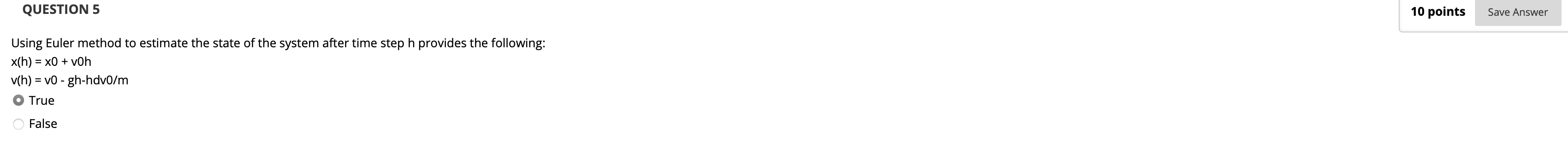## Week V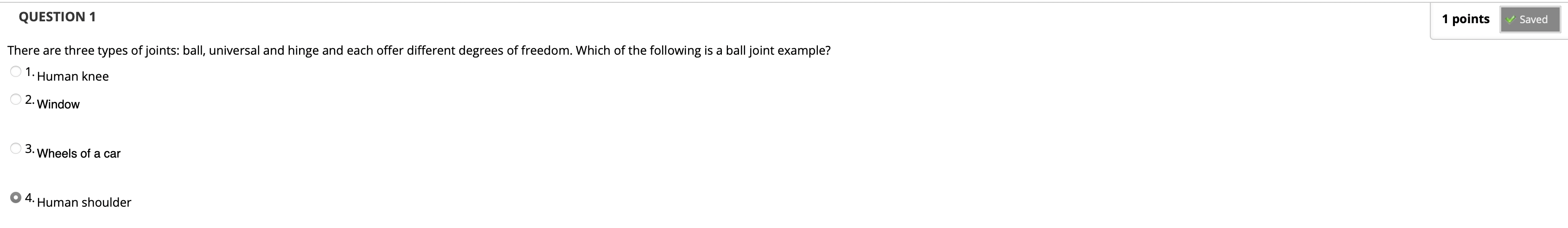hinge joint: 1 degree of freedom, (therefore when forming a hinge joint you need to decrease for 5 dofs)

ball joint: 3 degree of freedom (rotational), (therefore when forming a ball joint you need to decerase for 3 dofs)

universal joint: 2 degree of freedom (also rotational), (therefore when forming a universal joint you need to decrease for 4 dofs)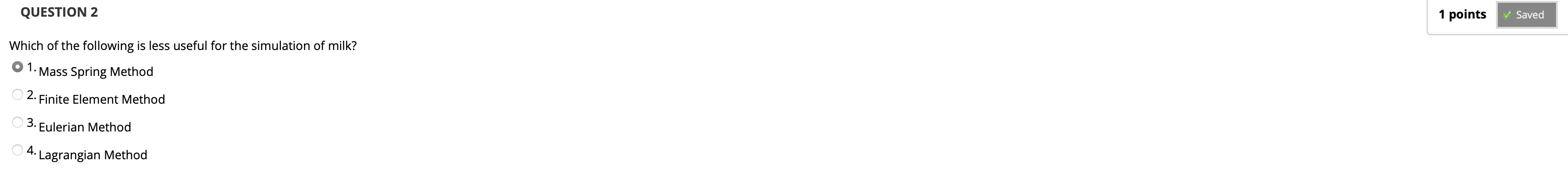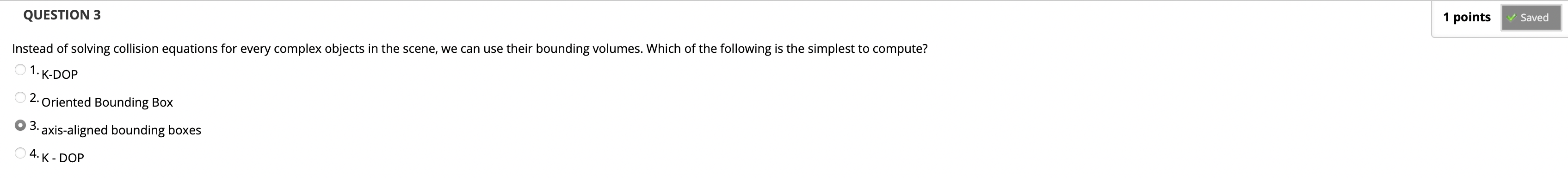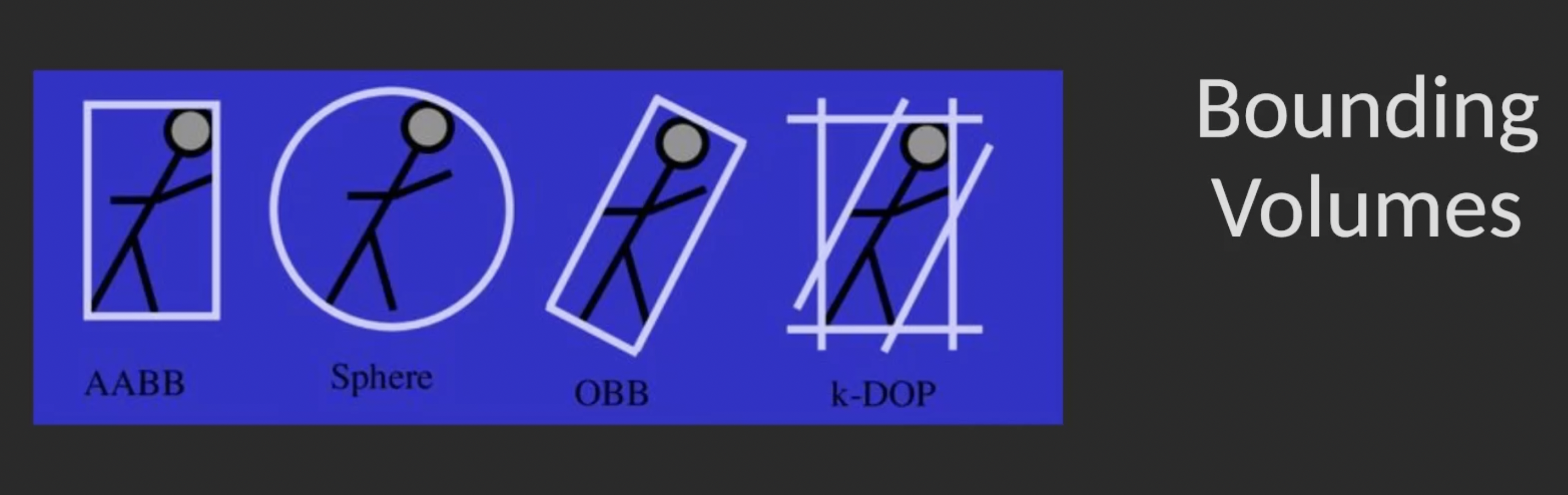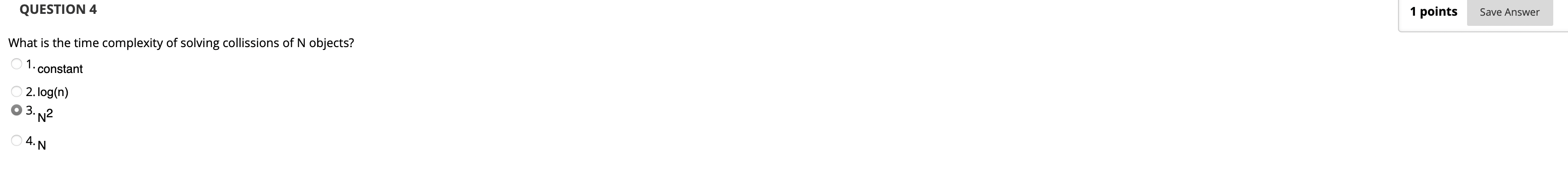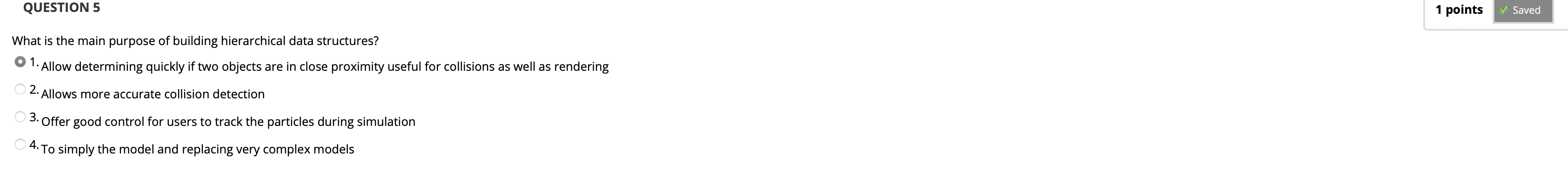## Week VI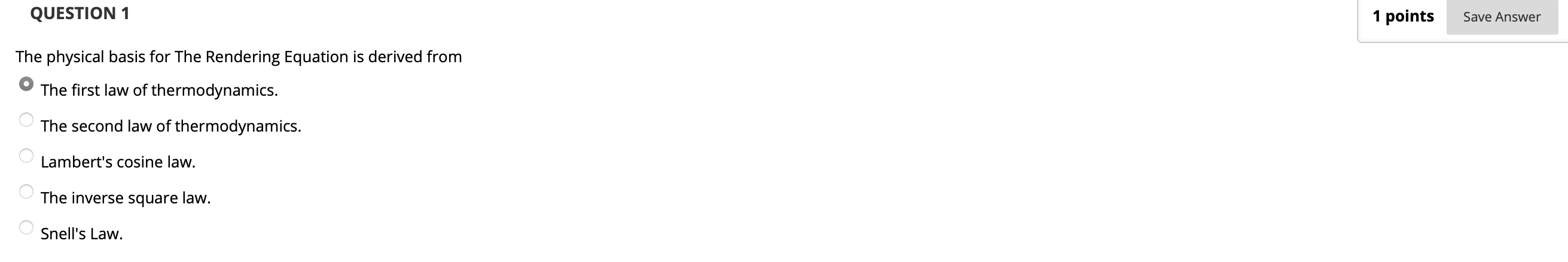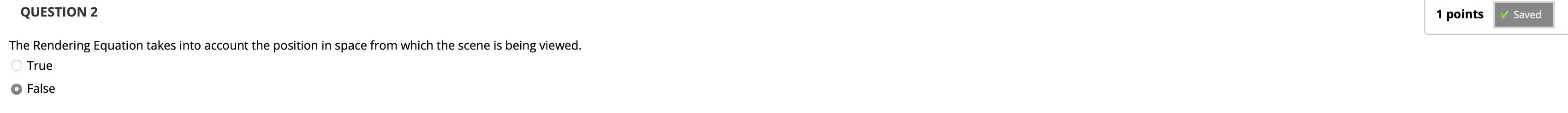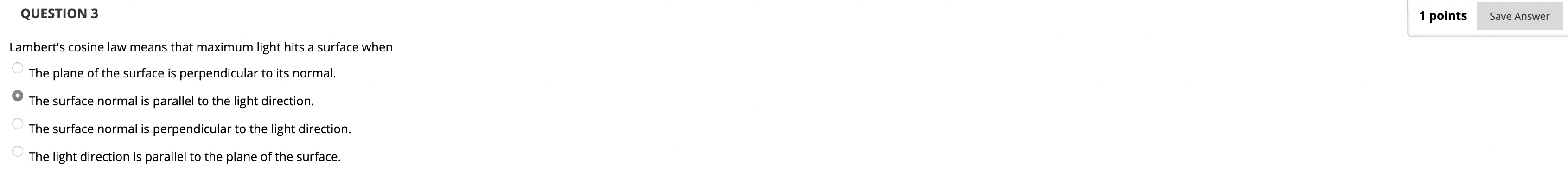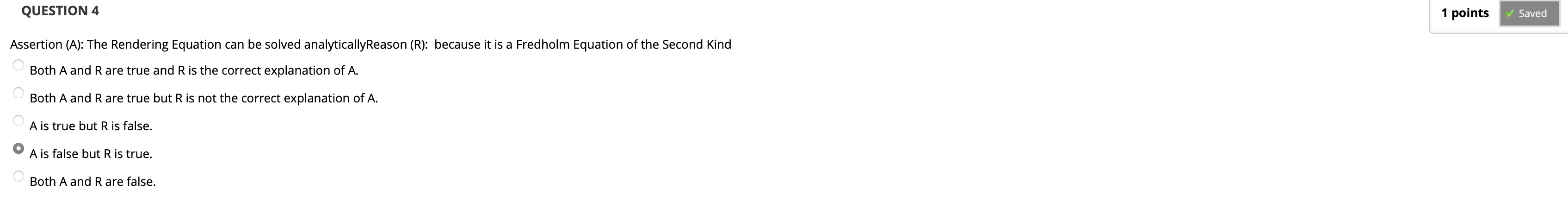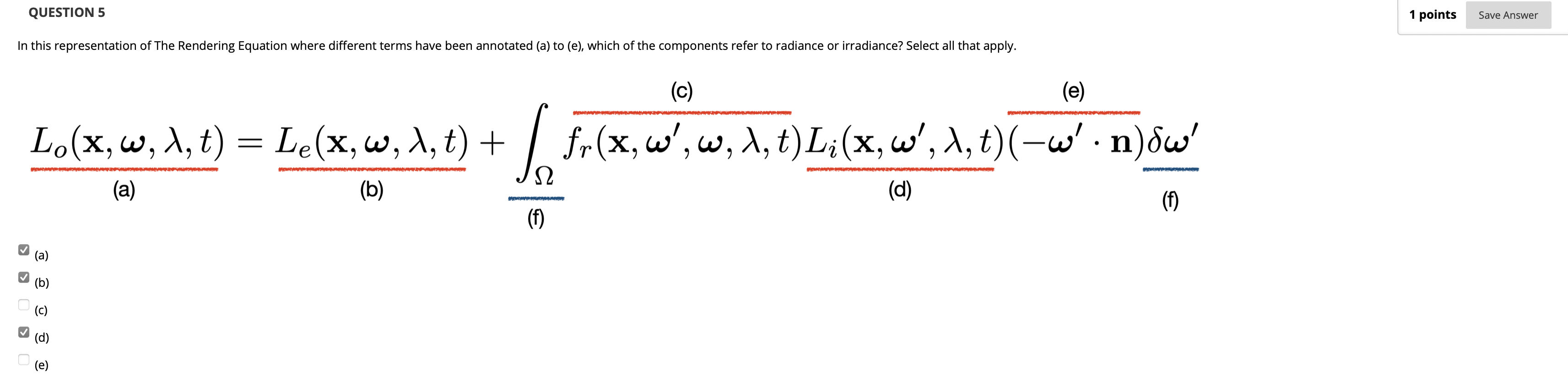a) 为我们从观察点所观测的, 目标点发散出的能量辐射 (Radiance), b) 为该点自身发散出的能量辐射 (Radiance), d 为不同方向上射入目标点的能量辐照 (Irradiance).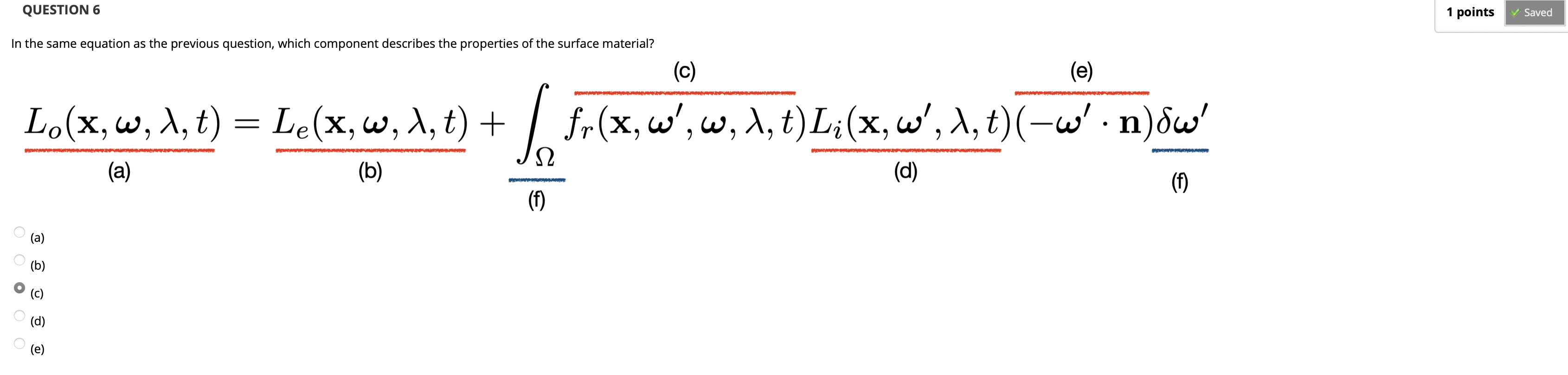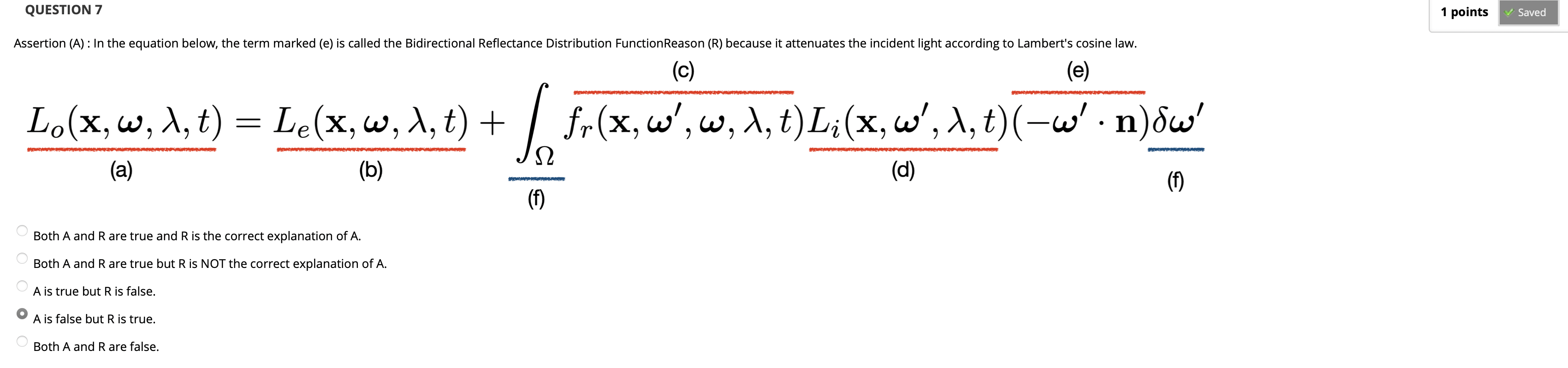## Week VII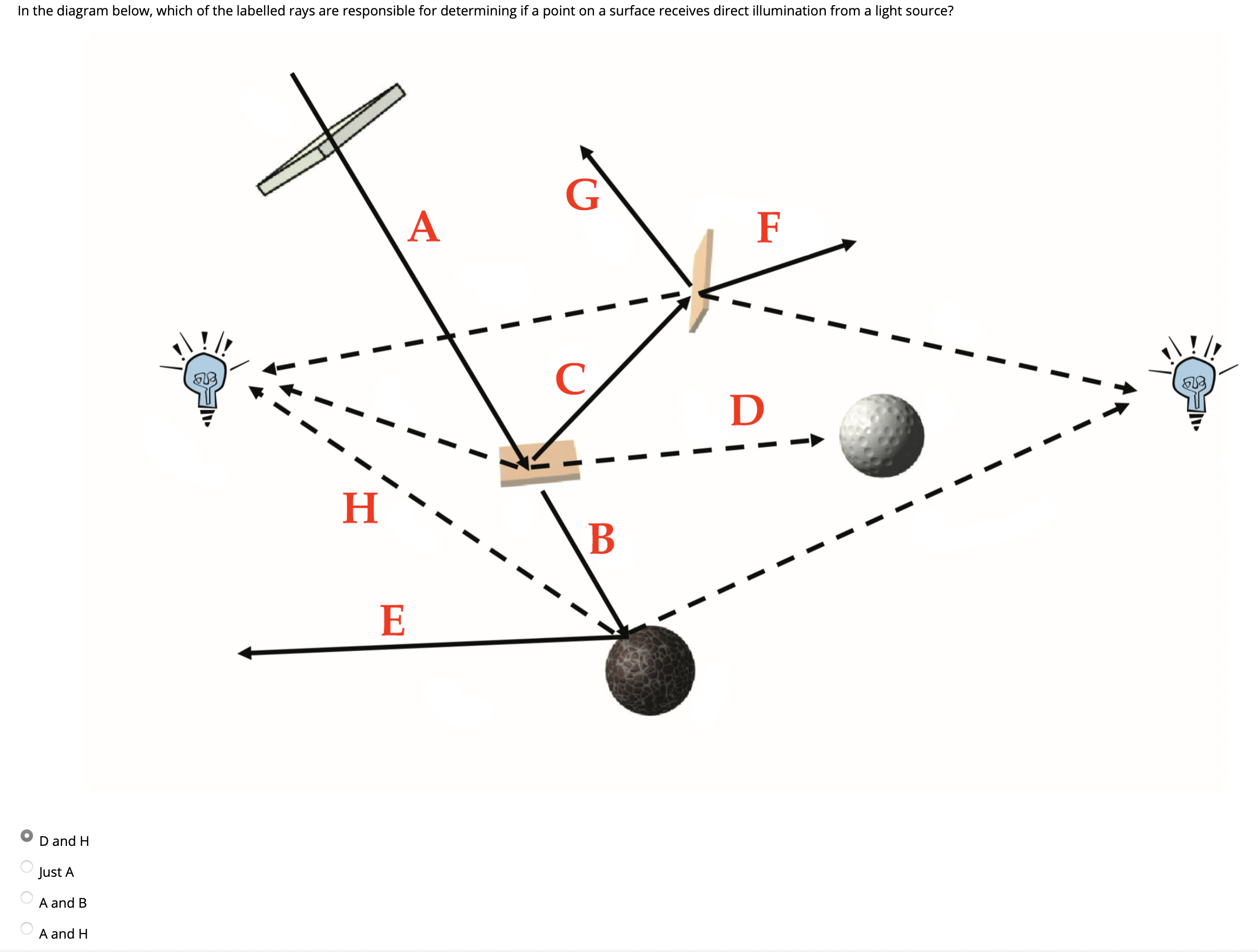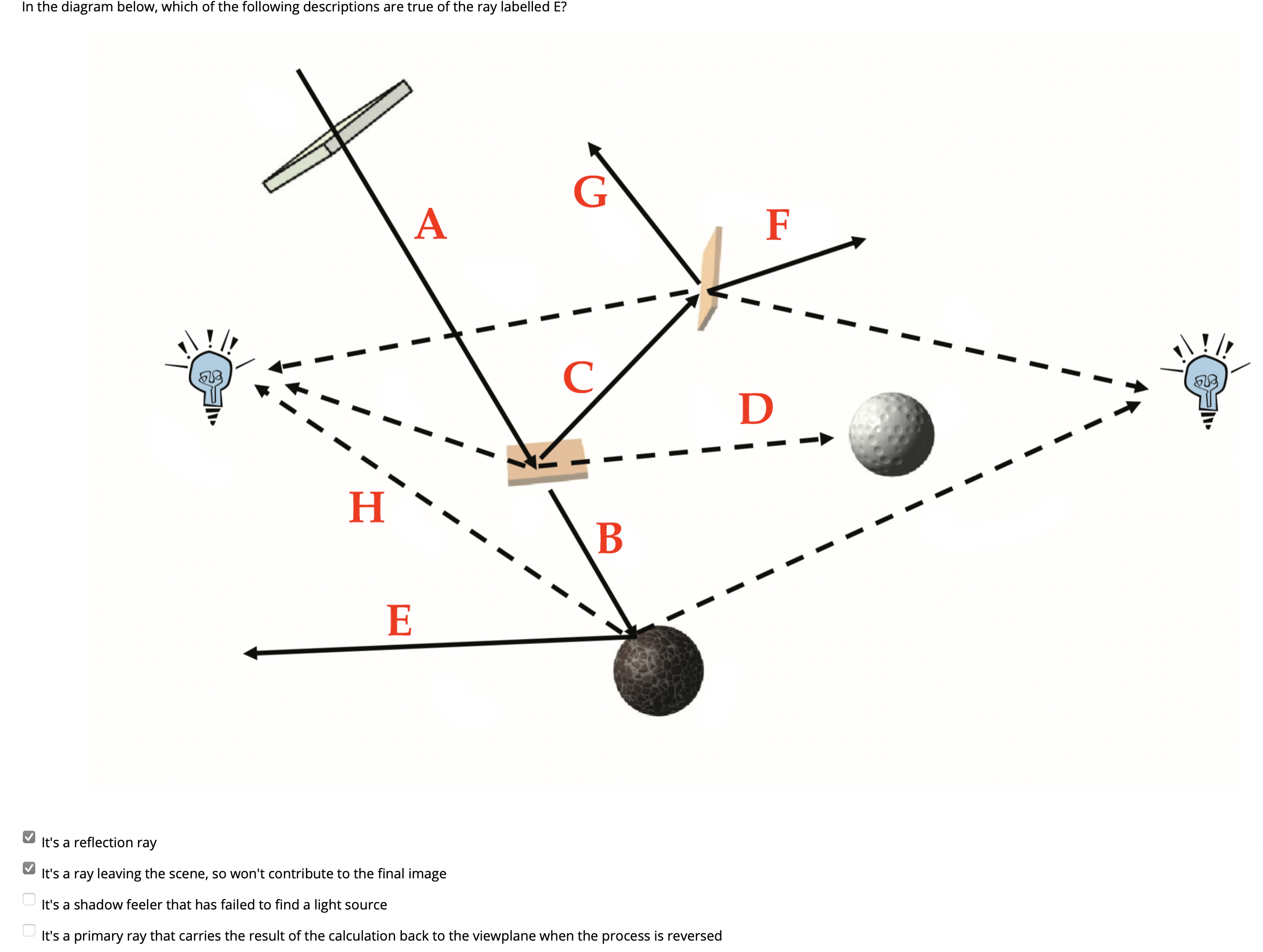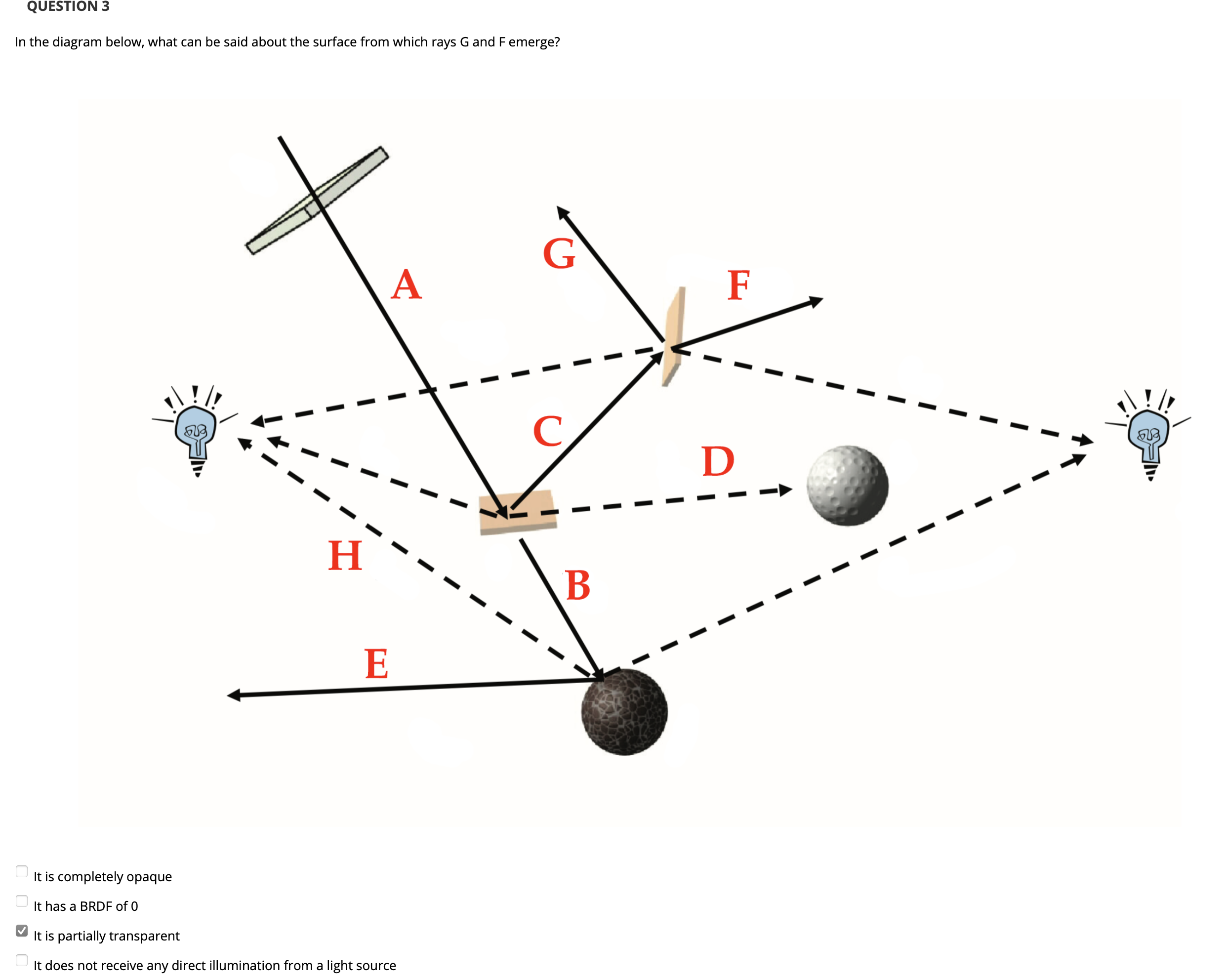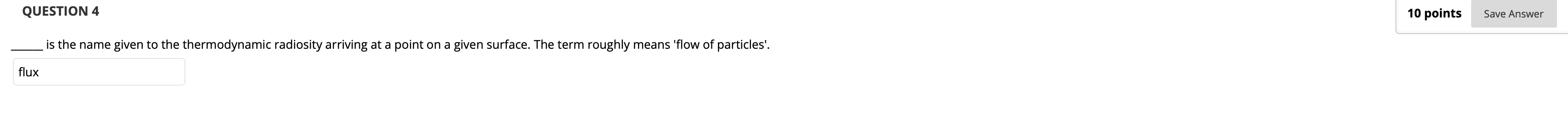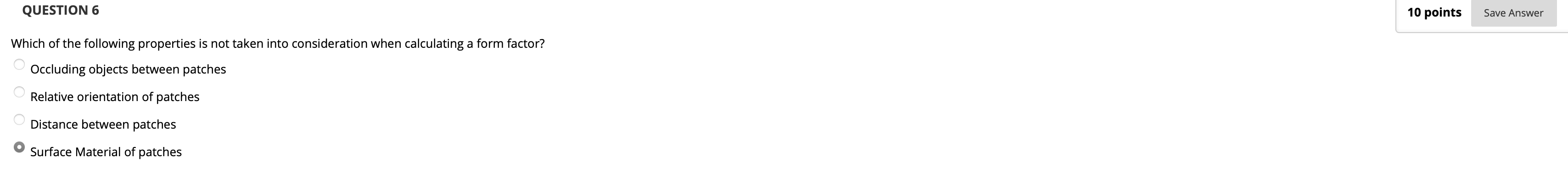Form Factor 是描述两个面之间是否存在 遮挡 的量, 在确定 Form Factor 时显然考虑物体表面的材质不会有任何实际意义.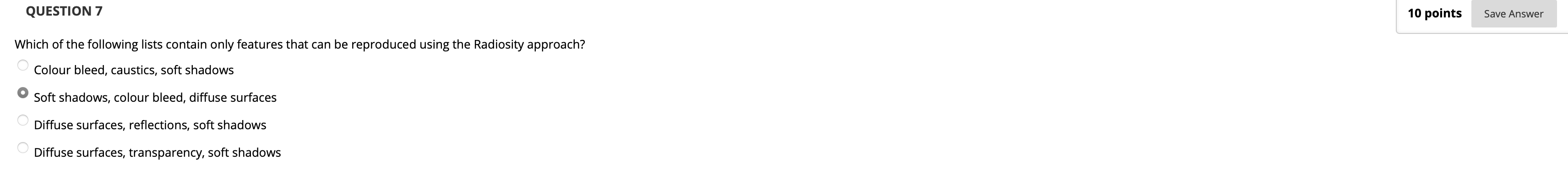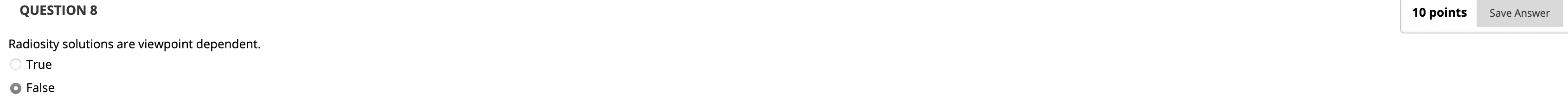## Week VIII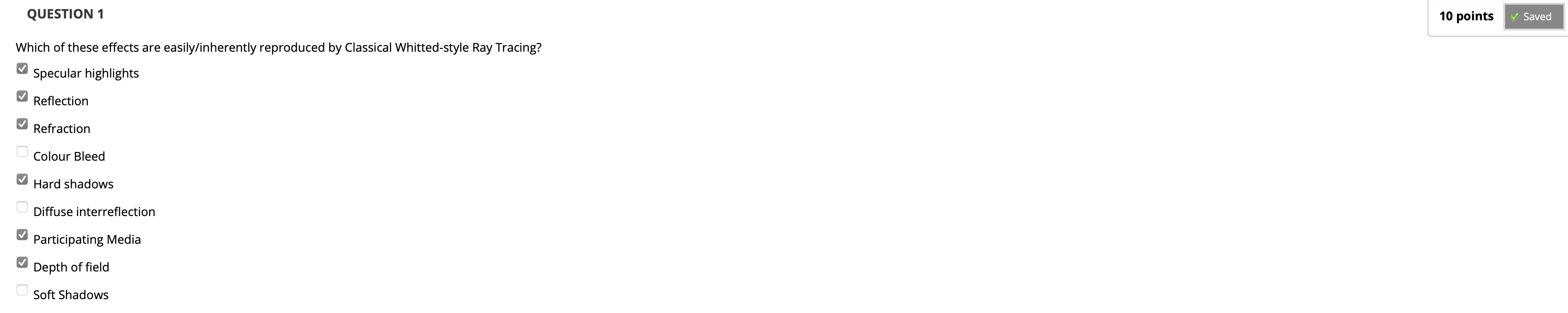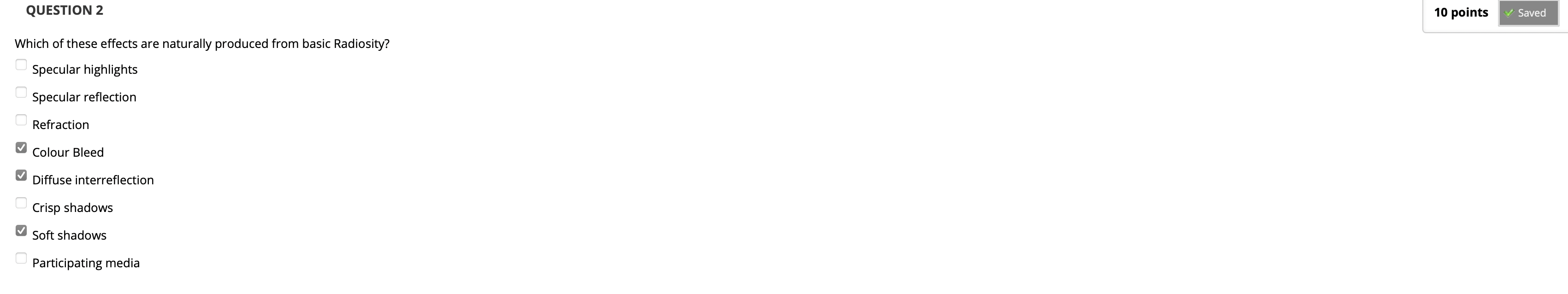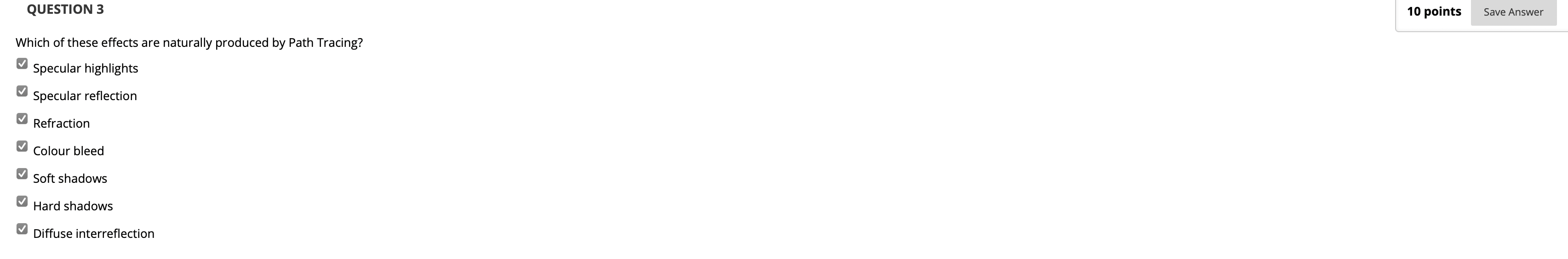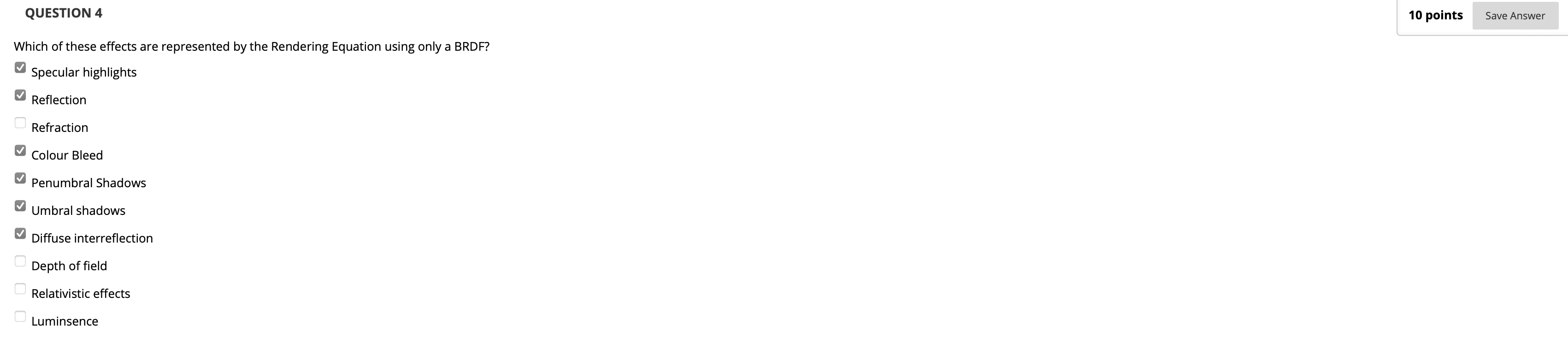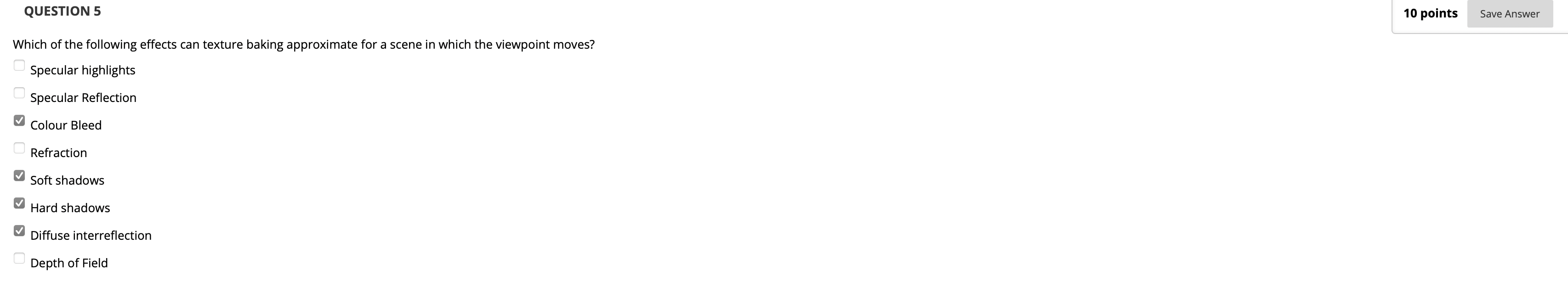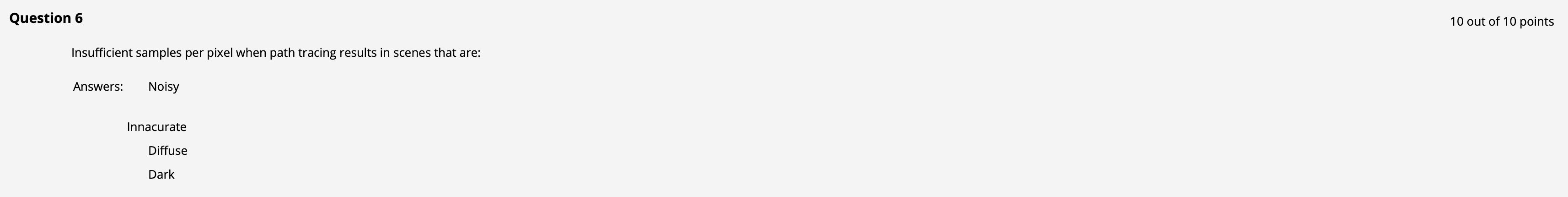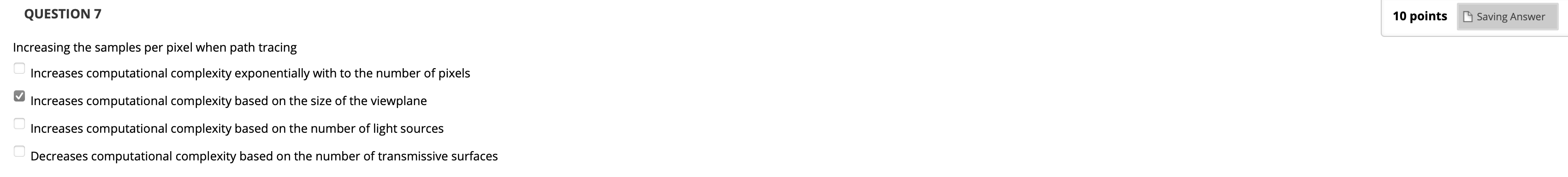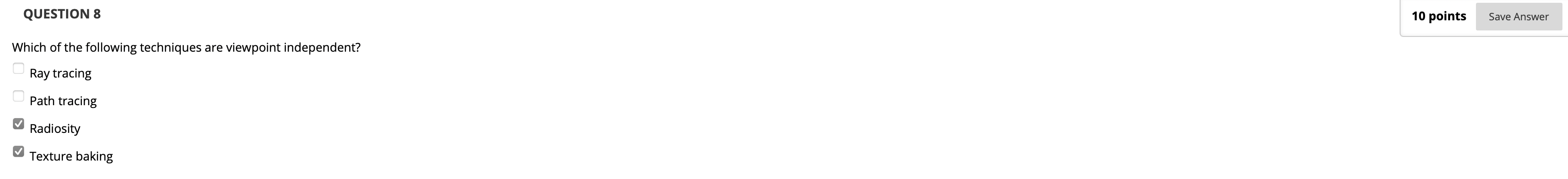## Week IX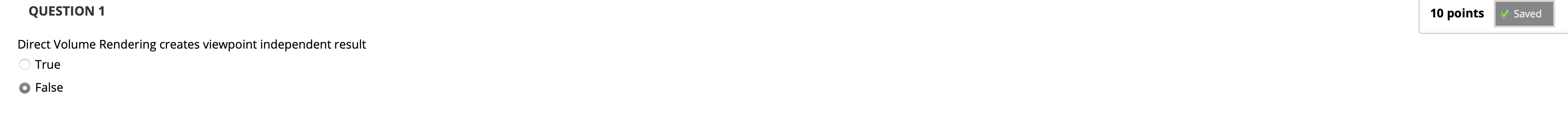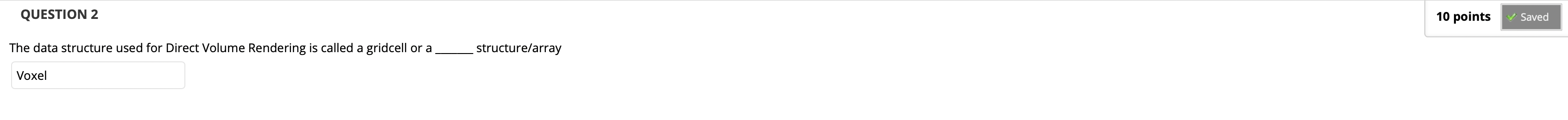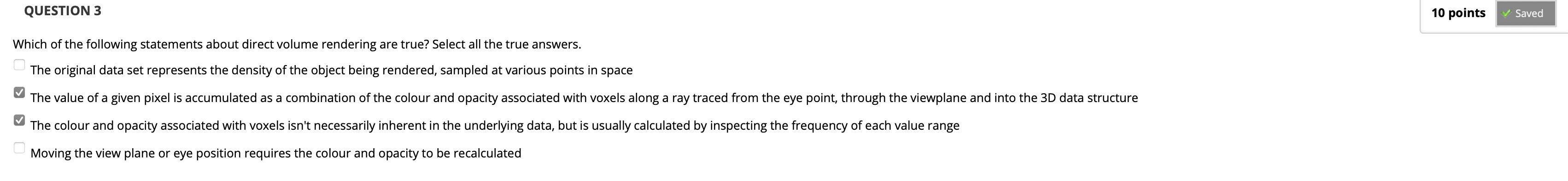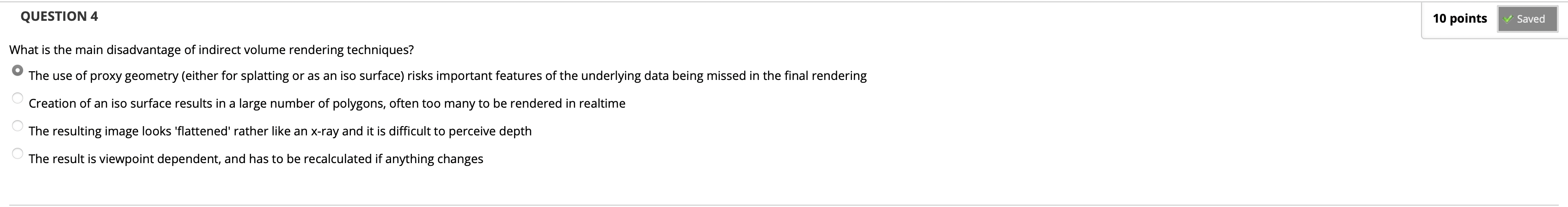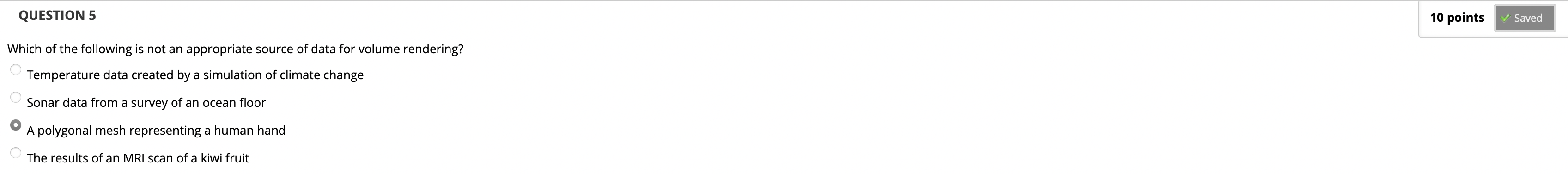## Week X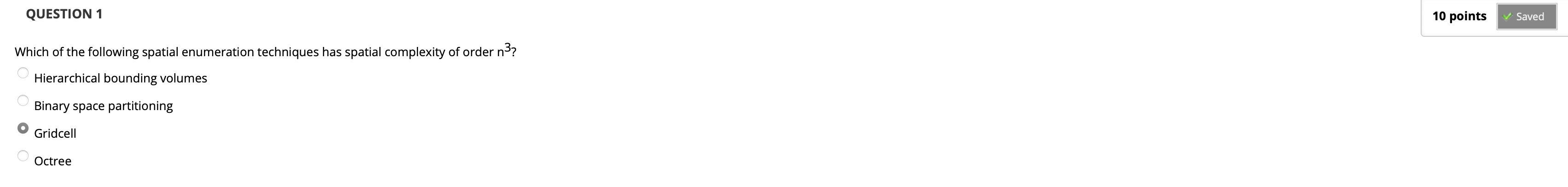Gridcell 背后的数据结构是 $3$ 维数组.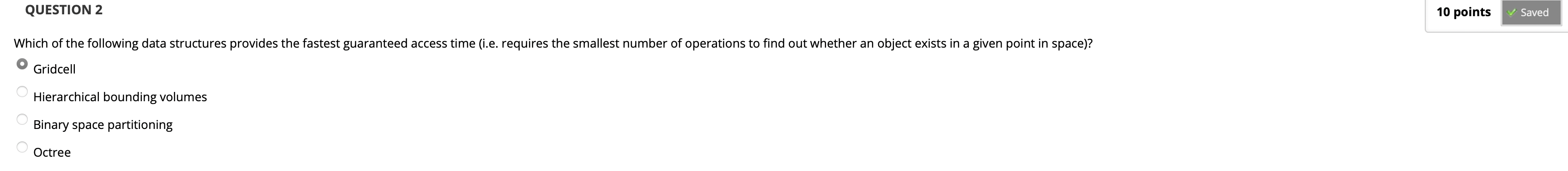$3$ 维数组的访问时间复杂度是 $O(1)$.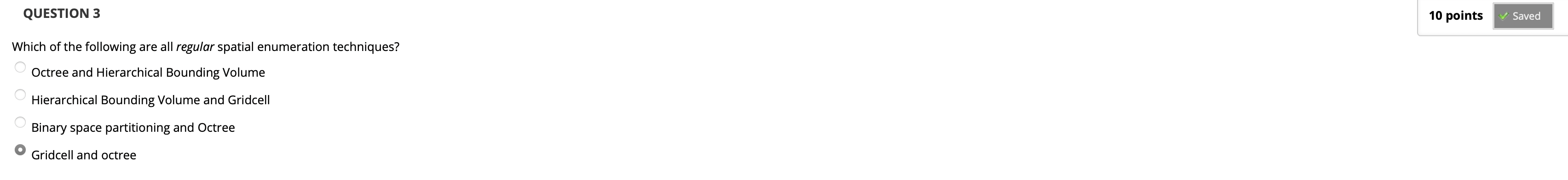GridcellOctree 的索引构建过程都是基于给定规则的.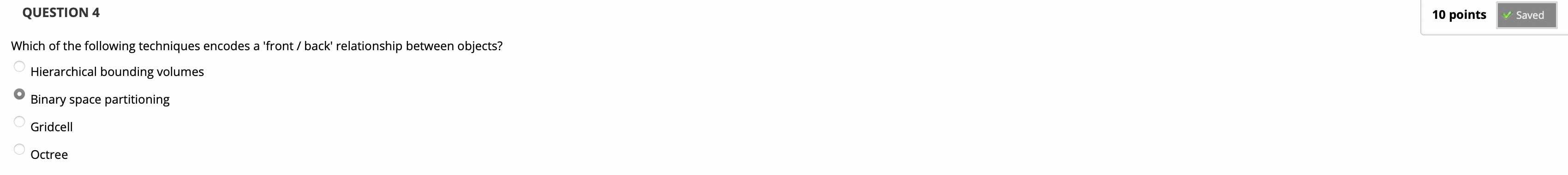Binary Space Partitioning 分划可以保留空间中的相对前后, 上下和左右关系.